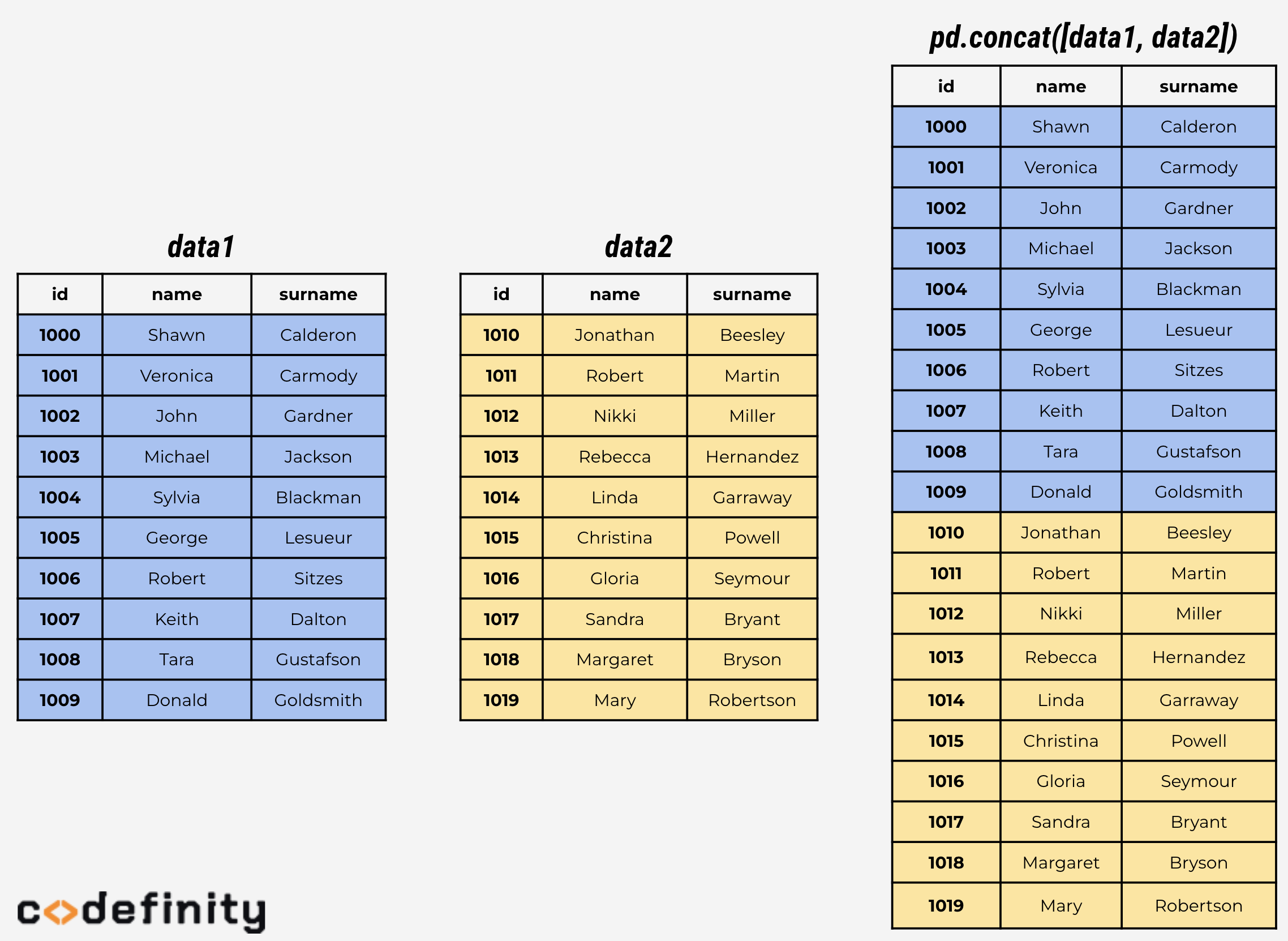Course Content

Data Manipulation using pandasConcatenation

Great! You've learned different ways of joining columns. Can we join two dataframes by rows? Surely, we can.

To join two dataframe, use the `.concat()` method of `pandas`, passing list of dataframes you want to join as the parameter. To perform this operation, both dataframes must have the same column names. Let's use splitted into two parts one of the dataframes used before and perform rows joining.

Note that `.concat()` is method of `pandas`, unlike the `.merge()` method. So you need to use `pd.concat()`.Section 5.

Chapter 6